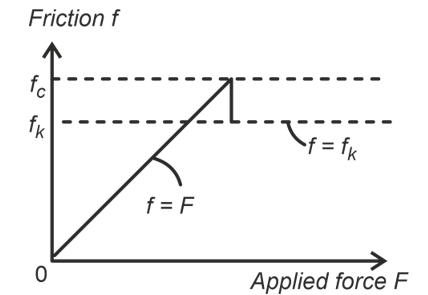# Short Notes and Formulas of class 8 science chapter-Friction

May 13, 2022, 16:45 IST

## Class- 8 science chapter-Friction

### Friction Force

The force acting along the two surfaces in contact which opposes the motion or the tendency of motion of one body over the other is known as the force of friction or frictional force. It acts on both the surfaces in contact.

### Reason behind friction

Force of friction arises due to the force of adhesion at the point of contact. Adhesion is the force of attraction between molecules (material) of different kind. When two rough surfaces come in contact, many contact points are formed. The atoms or molecules present at such points of contact attracts due to adhesive forces which opposes motion of one body over other.

There are two areas of contact- apparent area of contact & actual area of contact.

### Factors Affecting Friction

• Friction depends on the following:
• Nature of surfaces.
• Normal forces (friction forces is directly proportional to normal force between the contact surfaces)
•  Actual area of contact and the apparent increases with increase in actual area of contact)

### Types of friction

Friction is of three types:

• Static friction
• Sliding friction
• Rolling friction

Static friction is the friction acting when one body is not moving over other body but there is a tendency to move.

Sliding friction is the friction acting when one body is moving over other body. Sliding friction is also known as kinetic frictionRolling friction acts when body rolls over another rough body.

### Friction due to liquid and gases

When a solid moves in a liquid or gas its surface experiences a frictional force. This frictional force exerted by fluids is also known as drag. It is established that fsolid >fliquid >fgas

The shape of the body around which fluid (liquid or gas) can easily flow, offering minimum friction is called as streamlined flow.

### Effects of Friction

• Friction opposes motion of body over other body.
• Friction produces Heat (rubbing of surfaces leads to evolution of heat)

Do solve NCERT questions with the help of NCERT solutions for class 8 Science.

Find Pdf of chapter-Friction  having Important short notes of chapter